# How To Calculate Percentages In Excel (Plus 5 Helpful Tips)

Finding percentages helps us to understand how values are increasing or decreasing over time (among other things).  Microsoft Excel can find the percentages for any pair of values (numerator and denominator, or dividend and divisor) you choose as inputs.

So, how do you find percentages in Excel?  To find percentages in Excel, use the “/” symbol (division). Each input is a number or a cell: the numerator (dividend) is before the “/” symbol, and the denominator (divisor) is after the “/” symbol. For example, the formula “=4/80” gives the value 0.05 (as a decimal) or 5% (as a percentage).

Of course, we have the option to display calculated quotients as either decimals or percentages. To convert a decimal to a percentage, we move the decimal point two places right (so the decimal 0.015 is 1.5 percent).

In this article, we’ll talk about how to find percentages in Excel.  We’ll also look at some examples to make the concept clear.

Let’s get started.

## How To Find Percentages In Excel

The easiest way to find a percentage in Excel is to use the “/” symbol.  This symbol is really a division function that takes two arguments (inputs): one before the “/” symbol, and one after the “/” symbol:

• First Input: the numerator of the fraction (dividend) comes before the “/” symbol.
• Second Input: the denominator of the fraction (divisor) comes after the “/” symbol.

Each input is either:

• A value (such as 5, 2.7, or 0.08)
• A cell that contains a value (such as A2 or B15)

Note that the second input (the divisor) cannot be zero.  Otherwise, we would get a zero denominator, which causes the “#DIV/0!” error.

The formula for percent in Excel has the form:

• Numerator / Denominator

Or

• Dividend / Divisor

Either way, we are calculating a decimal from a fraction.  We can then display this decimal as a percentage (by moving the decimal point two places to the right).

### Where Is Percent Style In Excel?

The “percent” style to display percentages in Excel is on the “Home” tab at the top of the window.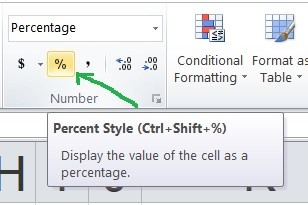The percent style button in Excel is on the “Home” tab, and it looks like the % symbol.

#### Example: How To Find A Percentage In Excel

Let’s say we have the following data table:

If we want to calculate the percentage of athletes in this group who are over 50, we need to use the division symbol “/”.

The first input (before the “/” symbol) is 17, which is the number of athletes over 50.

The second input (after the “/” symbol) is 50, which is the total number of athletes.

So, our percentage formula would be:

• =17/50”

which would show up as a value of 0.34 in Excel.

Note: you may see fewer decimal places (for example, 0.3).  In that case, you can display more decimal places by using the “Increase Decimal” button on the “Home” tab in Excel (at the top of the window).

To display the 0.34 value as a percentage:

• Select the cell that contains the “=17/50” division calculation.
• Select the “Home” tab in Excel (at the top of the window)
• Click on the “Percent Style” button in the “Home” tab (at the top of the window).

Alternatively, you can use keyboard shortcuts with these steps:

• Select the cell that contains the “=17/50” division calculation.
• Press the key combination “Ctrl + Shift + 5”.

You will now see 34% displayed, instead of 0.34.

### How To Find Percent Of A Number In Excel

To find percent of a number in Excel, you need to multiply two values together:

• The percentage, as a decimal (this can be a value or in a cell).
• The number (this can be a value or in a cell).

Remember the rule for converting percentages to decimals: we move the decimal point two places to the left.  So, 12% would be 0.12 as a decimal.

Let’s look at some examples to see how this works.

#### Example 1: How To Find 10 Percent Of A Number In Excel

If we want to find 10 percent of 60 In excel, we could do it in several ways.

One way is to use the formula:

• =0.1*60

in any available cell, since the “*” symbol is multiplication.

another way is to use the formula:

• =PRODUCT(0.1, 60)

in any available cell.

For both of the methods above, we used values as both arguments.  We can also use cells that contain values.

For example, let’s say that we have the value 0.1 (which is the decimal for 10%) in cell A1, as shown below.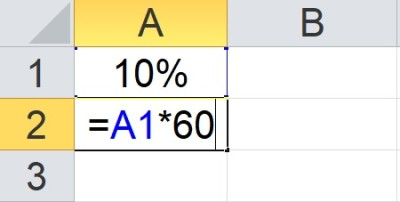The formula in cell A2 (=A1*60) uses the percentage in cell A1 (10%) and a value of 60 to find 10 percent of 60.

We can find 10 percent of 60 with the formula

• =A1*60

or

• =PRODUCT(A1, 60)

If we also have the value 60 in the cell A2, we can find 10 percent of 60 with the formula

• =A1*A2

or

• =PRODUCT(A1, A2)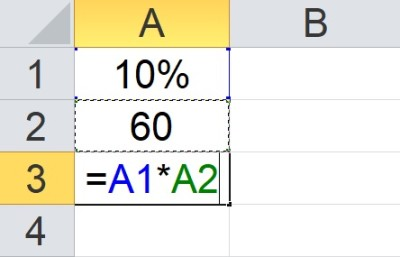The formula in cell A3 (=A1*A2) uses the percentage in cell A1 (10%) and the value in cell A2 (60) to find 10 percent of 60.

#### Example 2: How To Find 15 Percent Of A Number In Excel

To find 15 percent of a number in Excel, we would use steps similar to the previous example.  However, we would use the decimal 0.15 for 15 percent.

So, if we want 15 percent of 200, we could take these steps:

• Enter 0.15 in cell A1
• Enter 200 in cell A2
• Enter the formula “=A1*A2” in cell A3

We will see the value 30 in cell A3, which is 15 percent of 200 (0.15*200 = 30).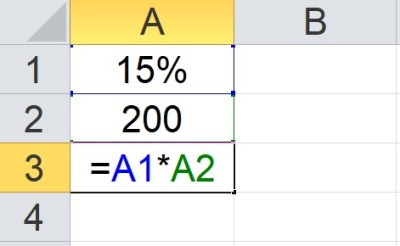The formula in cell A3 (=A1*A2) uses the percentage in cell A1 (15%) and the value in cell A2 (200) to find 15 percent of 200.

#### Example 3: How To Find 75 Percent Of A Number In Excel

To find 75 percent of a number in Excel, we would use steps similar to the previous example.  However, we would use the decimal 0.75 for 75 percent.

So, if we want 75 percent of 300, we could take these steps:

• Enter 0.75 in cell A1
• Enter 300 in cell A2
• Enter the formula “=A1*A2” in cell A3

We will see the value 225 in cell A3, which is 75 percent of 300 (0.75*300 = 225).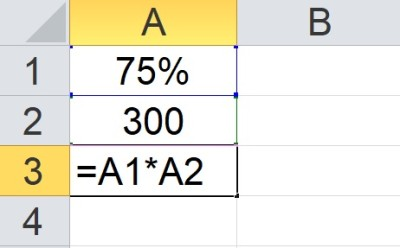The formula in cell A3 (=A1*A2) uses the percentage in cell A1 (75%) and the value in cell A2 (300) to find 75 percent of 300.

#### Example 4: How To Find 2 Percent Of A Number In Excel

To find 2 percent of a number in Excel, we would use steps similar to the previous example.  However, we would use the decimal 0.02 for 2 percent (don’t forget the zero after the decimal place – remember that 0.2 is 20 percent, not 2 percent!)

So, if we want 2 percent of 800, we could take these steps:

• Enter 0.02 in cell A1
• Enter 800 in cell A2
• Enter the formula “=A1*A2” in cell A3

We will see the value 16 in cell A3, which is 2 percent of 800 (0.02*800 = 16).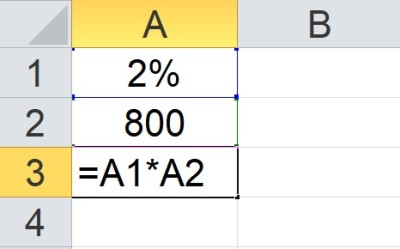The formula in cell A3 (=A1*A2) uses the percentage in cell A1 (2%) and the value in cell A2 (800) to find 2 percent of 800.

### How To Calculate Percentage Increase Or Decrease In Excel

To calculate percentage increase (or decrease) in Excel, we need to know which value is the “old” (original) value and which one is the “new” (increased or decreased) value.

We use the general formula:

• Percentage Increase = (new – old) / old

*Note: if the percentage is negative (new is less than old), then it would be a percentage decrease.

Here are the steps in words:

• Find out how much the value has changed (new minus old).  This is the numerator.
• Divide by the old value (which is the denominator).

In Excel, if we have the old value in cell A1 and the new value in cell A2, then we can calculate the percentage change with the following formula in cell A3:

• (A2 – A1)/A1

To display this value as a percentage, we use the same steps as before:

• Select cell A3 (or whatever cell contains the percentage calculation).
• Press the key combination “Ctrl + Shift + 5”.

#### Example 1: How To Calculate Percentage Increase In Excel

Let’s say we have an old (original) value of 200 in cell A1 and a new value of 260 in cell A2.

Using the formula above in cell A3, we get 0.3 (a 30 percent increase).

We can verify this as follows:

• a change from 200 to 260 is an increase of 60
• an increase of 60 divided by the original value of 200 is 60/200 = 0.3, which is a 30% increase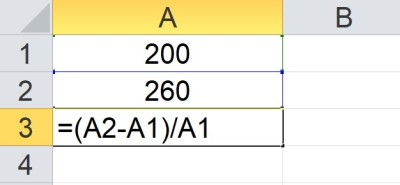The formula in cell A3 =(A2-A1)/A1 uses the percentage change formula to find a 30% increase from 200 to 260.

#### Example 2: How To Calculate Percentage Decrease In Excel

Let’s say we have an old (original) value of 400 in cell A1 and a new value of 320 in cell A2.

Using the formula above in cell A3, we get -0.2 (a 20 percent decrease).

We can verify this as follows:

• a change from 400 to 320 is an decrease of 80
• a decrease of 80 divided by the original value of 400 is 80/400 = 0.2, which is a 20% decrease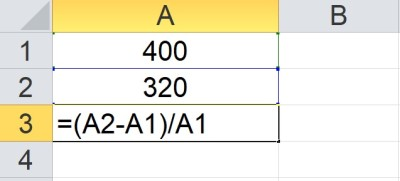The formula in cell A3 =(A2-A1)/A1 uses the percentage change formula to find a 20% decrease from 400 to 320.

### How To Add Or Subtract Percents In Excel

There are two basic ways to add percents in Excel.

One method is to calculate the percentage of the original value as a number and then add that number to the original value.

If the original value is in cell A1 and the percent is in cell A2, then we need two cells for calculations:

• In cell A3, we use the formula =A1*A2 (this calculates the percentage of the original value)
• In cell A4, we use the formula =A1 + A3  (this calculates the original value plus the percentage)

*Note: to subtract percents, we use subtraction (the formula in cell A4 would be A1 – A3 instead of A1 + A3).

A second method is to calculate the percentage first and then multiply by the original value.

If the original value is in cell A1 and the percent is in cell A2, then we need only one cell for calculations:

• In cell A3, we use the formula =A1 + A1*A2, or =A1(1 + A2)

*Note: to subtract percents, we use subtraction (the formula in cell A3 would be A1 – A1*A2, or =A1*(1 – A2). Otherwise, we need to enter a negative percentage in cell A2.

#### Example 1: How To Add 20 Percent In Excel

Let’s say we have an original value of 500 in cell A1 and 23% in cell A2.

Using the shortcut formula above in cell A3, we get 615 (an increase of 115).

We can verify this as follows:

• 23% of 500 is 115
• An increase of 115 from 500 is 615

#### Example 2: How To Add 7.5 Percent In Excel

Let’s say we have an original value of 600 in cell A1 and 7.5% in cell A2.

Using the shortcut formula above in cell A3, we get 654 (an increase of 54).

We can verify this as follows:

• 7.5% of 600 is 45
• An increase of 45 from 600 is 645

#### Example 3: How To Subtract 10 Percent In Excel

Let’s say we have an original value of 60 in cell A1 and -20% in cell A2.

Using the shortcut formula above in cell A3, we get 48 (a decrease of 12).

We can verify this as follows:

• 20% of 60 is 12
• An decrease of 12 from 60 is 48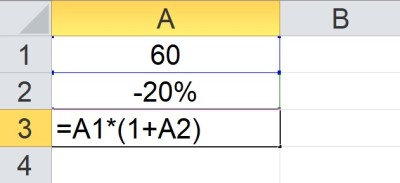The formula in cell A3 = A1*(1+A2) uses the percentage increase formula to find a 20% decrease from 60 to 48.

#### Example 4: How To Subtract 15 Percent In Excel

Let’s say we have an original value of 80 in cell A1 and -15% in cell A2.

Using the shortcut formula above in cell A3, we get 68 (a decrease of 12).

We can verify this as follows:

• 15% of 80 is 12
• An decrease of 12 from 68 is 68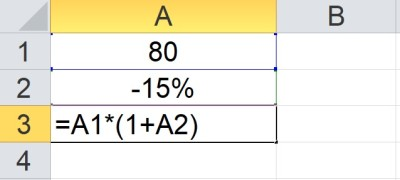The formula in cell A3 = A1*(1+A2) uses the percentage increase formula to find a 15% decrease from 80 to 68.

## Conclusion

Now you know how to find quartiles in Excel, what they mean, and what they can tell you about a data set.  You also know how to find the IQR (interquartile range) by subtracting Q1 from Q3 (IQR = Q3 – Q1).